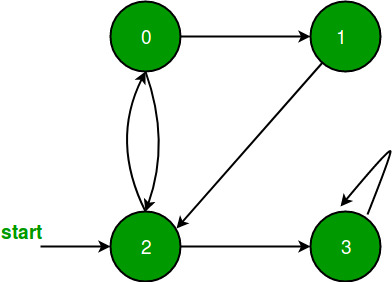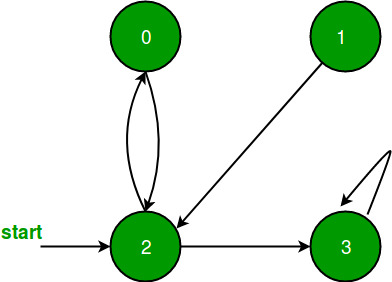# BFS for Disconnected Graph

• Difficulty Level : Easy
• Last Updated : 17 Jun, 2022

In the previous post, BFS only with a particular vertex is performed i.e. it is assumed that all vertices are reachable from the starting vertex. But in the case of a disconnected graph or any vertex that is unreachable from all vertex, the previous implementation will not give the desired output, so in this post, a modification is done in BFS.All vertices are reachable. So, for the above graph, simple BFS will work.As in the above graph vertex 1 is unreachable from all vertex, so simple BFS wouldn’t work for it.

```Just to modify BFS, perform simple BFS from each
unvisited vertex of given graph.```

Following is the code when adjacency matrix representation is used for the graph.

## C++

 `// C++ implementation of modified BFS for adjacency matrix``// representation``#include ``#include ``using` `namespace` `std;``void` `printBFS(``int``** edges, ``int` `V, ``int` `start, ``int``* visited);``void` `BFSHelper(``int``** edges, ``int` `V);``void` `addEdge(``int``** edges, ``int` `f, ``int` `s);` `void` `addEdge(``int``** edges, ``int` `f, ``int` `s) { edges[f][s] = 1; }``void` `printBFS(``int``** edges, ``int` `V, ``int` `start, ``int``* visited)``{``    ``if` `(V == 0)``        ``return``;``    ``queue<``int``> BFS;``    ``BFS.push(start);``    ``visited[start] = 1;``    ``while` `(!BFS.empty()) {``        ``int` `data = BFS.front();``        ``BFS.pop();``        ``cout << data << ``" "``;``        ``for` `(``int` `i = 0; i < V; i++) {``            ``if` `(edges[data][i] == 1) {``                ``if` `(visited[i] == 0) {``                    ``BFS.push(i);``                    ``visited[i] = 1;``                ``}``            ``}``        ``}``    ``}``}` `void` `BFSHelper(``int``** edges, ``int` `V)``{``    ``if` `(V == 0)``        ``return``;``    ``int``* visited = ``new` `int``[V];``    ``for` `(``int` `i = 0; i < V; i++) {``        ``visited[i] = 0;``    ``}``    ``for` `(``int` `i = 0; i < V; i++) {``        ``if` `(visited[i] == 0) {``            ``printBFS(edges, V, i, visited);``        ``}``    ``}``}` `int` `main()``{``    ``int` `V = 5;``    ``int` `E = 6;``    ``if` `(E == 0) {``        ``for` `(``int` `i = 0; i < V; i++) {``            ``cout << i << ``" "``;``        ``}``        ``return` `0;``    ``}``    ``int``** edges = ``new` `int``*[V];``    ``for` `(``int` `i = 0; i < V; i++) {``        ``edges[i] = ``new` `int``[V];``        ``for` `(``int` `j = 0; j < V; j++) {``            ``edges[i][j] = 0;``        ``}``    ``}` `    ``addEdge(edges, 0, 4);``    ``addEdge(edges, 1, 2);``    ``addEdge(edges, 1, 3);``    ``addEdge(edges, 1, 4);``    ``addEdge(edges, 2, 3);``    ``addEdge(edges, 3, 4);` `    ``BFSHelper(edges, V);``    ``return` `0;``}`

## Java

 `// Java implementation of modified BFS for adjacency matrix``// representation` `import` `java.io.*;``import` `java.util.*;` `class` `GFG {``  ``static` `void` `addEdge(``int``[][] edges, ``int` `f, ``int` `s)``  ``{``    ``edges[f][s] = ``1``;``  ``}` `  ``static` `void` `printBFS(``int``[][] edges, ``int` `V, ``int` `start,``                       ``int``[] visited)``  ``{``    ``if` `(V == ``0``)``      ``return``;``    ``Queue BFS = ``new` `LinkedList();``    ``BFS.add(start);``    ``visited[start] = ``1``;``    ``while` `(!BFS.isEmpty()) {``      ``int` `data = BFS.poll();``      ``System.out.print(data + ``" "``);``      ``for` `(``int` `i = ``0``; i < V; i++) {``        ``if` `(edges[data][i] == ``1``) {``          ``if` `(visited[i] == ``0``) {``            ``BFS.add(i);``            ``visited[i] = ``1``;``          ``}``        ``}``      ``}``    ``}``  ``}` `  ``static` `void` `bfsHelper(``int``[][] edges, ``int` `V)``  ``{``    ``if` `(V == ``0``)``      ``return``;``    ``int``[] visited = ``new` `int``[V];``    ``for` `(``int` `i = ``0``; i < V; i++) {``      ``visited[i] = ``0``;``    ``}``    ``for` `(``int` `i = ``0``; i < V; i++) {``      ``if` `(visited[i] == ``0``) {``        ``printBFS(edges, V, i, visited);``      ``}``    ``}``    ``System.out.println();``  ``}` `  ``public` `static` `void` `main(String[] args)``  ``{``    ``int` `V = ``5``;``    ``int` `E = ``6``;``    ``if` `(E == ``0``) {``      ``for` `(``int` `i = ``0``; i < V; i++) {``        ``System.out.print(i + ``" "``);``      ``}``      ``System.out.println();``      ``System.exit(``0``);``    ``}``    ``int``[][] edges = ``new` `int``[V][V];``    ``for` `(``int` `i = ``0``; i < V; i++) {``      ``for` `(``int` `j = ``0``; j < V; j++) {``        ``edges[i][j] = ``0``;``      ``}``    ``}` `    ``addEdge(edges, ``0``, ``4``);``    ``addEdge(edges, ``1``, ``2``);``    ``addEdge(edges, ``1``, ``3``);``    ``addEdge(edges, ``1``, ``4``);``    ``addEdge(edges, ``2``, ``3``);``    ``addEdge(edges, ``3``, ``4``);` `    ``bfsHelper(edges, V);``  ``}``}` `// This code is contributed by cavi4762.`

## C#

 `// C# implementation of modified BFS for adjacency matrix``// representation` `using` `System;``using` `System.Collections.Generic;` `class` `Gfg {``  ``static` `void` `AddEdge(``int``[, ] edges, ``int` `f, ``int` `s)``  ``{``    ``edges[f, s] = 1;``  ``}` `  ``static` `void` `PrintBFS(``int``[, ] edges, ``int` `V, ``int` `start,``                       ``int``[] visited)``  ``{``    ``if` `(V == 0)``      ``return``;``    ``Queue<``int``> BFS = ``new` `Queue<``int``>();``    ``BFS.Enqueue(start);``    ``visited[start] = 1;``    ``while` `(BFS.Count > 0) {``      ``int` `data = BFS.Dequeue();``      ``Console.Write(data + ``" "``);``      ``for` `(``int` `i = 0; i < V; i++) {``        ``if` `(edges[data, i] == 1) {``          ``if` `(visited[i] == 0) {``            ``BFS.Enqueue(i);``            ``visited[i] = 1;``          ``}``        ``}``      ``}``    ``}``  ``}` `  ``static` `void` `BFSHelper(``int``[, ] edges, ``int` `V)``  ``{``    ``if` `(V == 0)``      ``return``;``    ``int``[] visited = ``new` `int``[V];``    ``for` `(``int` `i = 0; i < V; i++) {``      ``visited[i] = 0;``    ``}``    ``for` `(``int` `i = 0; i < V; i++) {``      ``if` `(visited[i] == 0) {``        ``PrintBFS(edges, V, i, visited);``      ``}``    ``}``    ``Console.WriteLine();``  ``}` `  ``static` `void` `Main(``string``[] args)``  ``{``    ``int` `V = 5;``    ``int` `E = 6;``    ``if` `(E == 0) {``      ``for` `(``int` `i = 0; i < V; i++) {``        ``Console.Write(i + ``" "``);``      ``}``      ``Console.WriteLine();``      ``Environment.Exit(0);``    ``}``    ``int``[, ] edges = ``new` `int``[V, V];``    ``for` `(``int` `i = 0; i < V; i++) {``      ``for` `(``int` `j = 0; j < V; j++) {``        ``edges[i, j] = 0;``      ``}``    ``}` `    ``AddEdge(edges, 0, 4);``    ``AddEdge(edges, 1, 2);``    ``AddEdge(edges, 1, 3);``    ``AddEdge(edges, 1, 4);``    ``AddEdge(edges, 2, 3);``    ``AddEdge(edges, 3, 4);` `    ``BFSHelper(edges, V);``  ``}``}` `// This code is contributed by cavi4762.`

Output

`0 4 1 2 3 `

Following is the code when adjacency list representation is used for the graph.

## C++

 `// C++ implementation of modified BFS``#include``using` `namespace` `std;` `// A utility function to add an edge in an``// directed graph.``void` `addEdge(vector<``int``> adj[], ``int` `u, ``int` `v)``{``    ``adj[u].push_back(v);``}` `// A utility function to do BFS of graph``// from a given vertex u.``void` `BFSUtil(``int` `u, vector<``int``> adj[],``            ``vector<``bool``> &visited)``{` `    ``// Create a queue for BFS``    ``list<``int``> q;`` ` `    ``// Mark the current node as visited and enqueue it``    ``visited[u] = ``true``;``    ``q.push_back(u);`` ` `    ``// 'i' will be used to get all adjacent vertices 4``    ``// of a vertex list::iterator i;`` ` `    ``while``(!q.empty())``    ``{``        ``// Dequeue a vertex from queue and print it``        ``u = q.front();``        ``cout << u << ``" "``;``        ``q.pop_front();`` ` `        ``// Get all adjacent vertices of the dequeued``        ``// vertex s. If an adjacent has not been visited,``        ``// then mark it visited and enqueue it``        ``for` `(``int` `i = 0; i != adj[u].size(); ++i)``        ``{``            ``if` `(!visited[adj[u][i]])``            ``{``                ``visited[adj[u][i]] = ``true``;``                ``q.push_back(adj[u][i]);``            ``}``        ``}``    ``}``}` `// This function does BFSUtil() for all``// unvisited vertices.``void` `BFS(vector<``int``> adj[], ``int` `V)``{``    ``vector<``bool``> visited(V, ``false``);``    ``for` `(``int` `u=0; u adj[V];` `    ``addEdge(adj, 0, 4);``    ``addEdge(adj, 1, 2);``    ``addEdge(adj, 1, 3);``    ``addEdge(adj, 1, 4);``    ``addEdge(adj, 2, 3);``    ``addEdge(adj, 3, 4);``    ``BFS(adj, V);``    ``return` `0;``}`

## Java

 `// Java implementation of modified BFS``import` `java.util.*;``public` `class` `graph``{``    ``//Implementing graph using HashMap``    ``static` `HashMap> graph=``new` `HashMap<>();` `    ``//utility function to add edge in an undirected graph``public` `static` `void` `addEdge(``int` `a,``int` `b)``{``    ``if``(graph.containsKey(a))``    ``{``        ``LinkedList l=graph.get(a);``        ``l.add(b);``        ``graph.put(a,l);``    ``}``    ``else``    ``{``        ``LinkedList l=``new` `LinkedList<>();``        ``l.add(b);``        ``graph.put(a,l);``    ``}``}` `//Helper function for BFS``public` `static` `void` `bfshelp(``int` `s,ArrayList visited)``{``    ``// Create a queue for BFS``    ``LinkedList q=``new` `LinkedList<>();``    ` `    ``// Mark the current node as visited and enqueue it``    ``q.add(s);``    ``visited.set(s,``true``);``    ` `    ``while``(!q.isEmpty())``    ``{``        ``// Dequeue a vertex from queue and print it``        ``int` `f=q.poll();``        ``System.out.print(f+``" "``);``        ` `        ``//Check whether the current node is``                ``//connected to any other node or not``        ``if``(graph.containsKey(f))``        ``{``        ``Iterator i=graph.get(f).listIterator();``        ` `        ``// Get all adjacent vertices of the dequeued``        ``// vertex f. If an adjacent has not been visited, ``        ``// then mark it visited and enqueue it``        ` `            ``while``(i.hasNext())``            ``{``                ``int` `n=i.next();``                ``if``(!visited.get(n))``                ``{``                ``visited.set(n,``true``);``                ``q.add(n);``                ``}``            ``}``        ``}``    ``}``    ` `}` `//BFS function to check each node``public` `static` `void` `bfs(``int` `vertex)``{``    ``ArrayList visited=``new` `ArrayList();``    ``//Marking each node as unvisited``    ``for``(``int` `i=``0``;i

## Python3

 `# Python3 implementation of modified BFS``import` `queue` `# A utility function to add an edge``# in an undirected graph.``def` `addEdge(adj, u, v):``    ``adj[u].append(v)` `# A utility function to do BFS of``# graph from a given vertex u.``def` `BFSUtil(u, adj, visited):` `    ``# Create a queue for BFS``    ``q ``=` `queue.Queue()``    ` `    ``# Mark the current node as visited``    ``# and enqueue it``    ``visited[u] ``=` `True``    ``q.put(u)``    ` `    ``# 'i' will be used to get all adjacent``    ``# vertices 4 of a vertex list::iterator i``    ` `    ``while``(``not` `q.empty()):``        ` `        ``# Dequeue a vertex from queue``        ``# and print it``        ``u ``=` `q.queue[``0``]``        ``print``(u, end ``=` `" "``)``        ``q.get()``    ` `        ``# Get all adjacent vertices of the``        ``# dequeued vertex s. If an adjacent``        ``# has not been visited, then mark``        ``# it visited and enqueue it``        ``i ``=` `0``        ``while` `i !``=` `len``(adj[u]):``            ``if` `(``not` `visited[adj[u][i]]):``                    ``visited[adj[u][i]] ``=` `True``                    ``q.put(adj[u][i])``            ``i ``+``=` `1` `# This function does BFSUtil() for all``# unvisited vertices.``def` `BFS(adj, V):``    ``visited ``=` `[``False``] ``*` `V``    ``for` `u ``in` `range``(V):``        ``if` `(visited[u] ``=``=` `False``):``            ``BFSUtil(u, adj, visited)` `# Driver code``if` `__name__ ``=``=` `'__main__'``:` `    ``V ``=` `5``    ``adj ``=` `[[] ``for` `i ``in` `range``(V)]` `    ``addEdge(adj, ``0``, ``4``)``    ``addEdge(adj, ``1``, ``2``)``    ``addEdge(adj, ``1``, ``3``)``    ``addEdge(adj, ``1``, ``4``)``    ``addEdge(adj, ``2``, ``3``)``    ``addEdge(adj, ``3``, ``4``)``    ``BFS(adj, V)` `# This code is contributed by PranchalK`

## C#

 `// C# implementation of modified BFS``using` `System;``using` `System.Collections.Generic;` `class` `Graph``{``    ``//Implementing graph using Dictionary``    ``static` `Dictionary<``int``,List<``int``>> graph =``            ``new` `Dictionary<``int``,List<``int``>>();` `//utility function to add edge in an undirected graph``public` `static` `void` `addEdge(``int` `a, ``int` `b)``{``    ``if``(graph.ContainsKey(a))``    ``{``        ``List<``int``> l = graph[a];``        ``l.Add(b);``        ``if``(graph.ContainsKey(a))``            ``graph[a] = l;``        ``else``            ``graph.Add(a,l);``    ``}``    ``else``    ``{``        ``List<``int``> l = ``new` `List<``int``>();``        ``l.Add(b);``        ``graph.Add(a, l);``    ``}``}` `// Helper function for BFS``public` `static` `void` `bfshelp(``int` `s, List visited)``{``    ``// Create a queue for BFS``    ``List<``int``> q = ``new` `List<``int``>();``    ` `    ``// Mark the current node as visited and enqueue it``    ``q.Add(s);``    ``visited.RemoveAt(s);``    ``visited.Insert(s,``true``);``    ` `    ``while``(q.Count != 0)``    ``{``        ``// Dequeue a vertex from queue and print it``        ``int` `f = q;``        ``q.RemoveAt(0);``        ``Console.Write(f + ``" "``);``        ` `        ``//Check whether the current node is``        ``//connected to any other node or not``        ``if``(graph.ContainsKey(f))``        ``{``        ` `        ``// Get all adjacent vertices of the dequeued``        ``// vertex f. If an adjacent has not been visited,``        ``// then mark it visited and enqueue it``        ` `            ``foreach``(``int` `iN ``in` `graph[f])``            ``{``                ``int` `n = iN;``                ``if``(!visited[n])``                ``{``                    ``visited.RemoveAt(n);``                    ``visited.Insert(n, ``true``);``                    ``q.Add(n);``                ``}``            ``}``        ``}``    ``}``    ` `}` `// BFS function to check each node``public` `static` `void` `bfs(``int` `vertex)``{``    ``List visited = ``new` `List();``    ` `    ``// Marking each node as unvisited``    ``for``(``int` `i = 0; i < vertex; i++)``    ``{``        ``visited.Insert(i, ``false``);``    ``}``    ``for``(``int` `i = 0; i < vertex; i++)``    ``{``        ``// Checking whether the node is visited or not``        ``if``(!visited[i])``        ``{``            ``bfshelp(i, visited);``        ``}``    ``}``}` `// Driver Code``public` `static` `void` `Main(String[] args)``{``    ``int` `v = 5;``    ``addEdge(0, 4);``    ``addEdge(1, 2);``    ``addEdge(1, 3);``    ``addEdge(1, 4);``    ``addEdge(2, 3);``    ``addEdge(3, 4);``    ``bfs(v);``}``}` `// This code is contributed by Rajput-Ji`

Output

`0 4 1 2 3 `